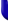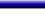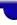Lighthouse3d.com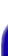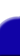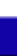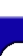Terrain Tutorial Index A TGA Library A Simple TGA Library TGA lib Source Height Maps Height Maps from Images Artificial Terrain Generation The Fault Algorithm Implementation Details Two Simple Variations The Circles Algorithm Smoothing Smoothing Matrix filters API details# Terrain Tutorial

## Implementation Details

In this section we'll see how to implement the algorithm presented in the previous section. We'll see how to compute the line that divides the terrain, and how to compute the displacement for each iteration.

Finding a Line Which Divides the Terrain to displace the heights

The first problem is how to create a random line which divides the terrain. A first approach could be to find a line crossing the origin with a random inclination. However this approach creates a terrain which is anti-symmetrical, i.e. if we have a mountain in one place, we'll have a depression on the other side. Therefore, to avoid this trap the line should not cross the origin in the general case.

The line formula is

```    ```
ax + bz = c

where  a*a + b*b = 1 and c >= 0
```
```

The formula above is know as the Normal Form. A simple way of obeying the restriction above is to consider

```    ```
a = sin(v)
b = cos(v)

where v is a random value.
```
```

Therefore a line, in its Normal Form, is an equation of two variables: v and c. So how do we compute the pair v,c so that the line actually divides the terrain?

Assuming that we're using the Normal Form, the distance from a point (x1,z1), to a line, ax+by-c=0, is defined by:

```    ```
dist = a*x1 + b*z1 - c
```
```

The distance will be negative for all points on one side of the line, zero for points in the line, and positive for points on the other side of the line.

Assuming that the terrain is centered on the origin the distance from the line to the origin, i.e. the point is (0,0), is defined solely by c.

```    ```
dist = a*0 + b*0 - c = -c
```
```

Therefore we only have to select a value for c which is smaller than the distance from the furthest point in the terrain to the origin. Assuming that the terrain has a width w, and a length l, then c should be defined in the interval [ -d/2, d/2 ], where d is defined as the square root of the sum of the squares of both w and l.

```    ```
d = sqrt(w*w + l*l)
```
```

The following code resumes what was explained above:

```    ```
v = rand();
a = sin(v);
b = cos(v);
d = sqrt(w*w + l*l);
// rand() / RAND_MAX gives a random number between 0 and 1.
// therefore c will be a random number between -d/2 and d/2
c = (rand() / RAND_MAX) * d - d/2;
```
```

Then, we iterate for each terrain point and displace its height, or y coordinate, by a certain amount. Points on one side of the line will have their height increased, whereas points on the other side will have their height decreased. The following pseudo code illustrates this iterative process

```    ```
for each point (tx,tz) in the terrain do {
if (a*tx + b*tz - c > 0)
height(tx,tz) += displacement;
else
height(tx,tz) -= displacement;
}
```
```

Another approach to find a line which divides the terrain, is when the line is constructed by selecting two random points from within the terrain. Assuming two points are given (x1,z1) and (x2,z2), the line equation is

```    ```
ax + bz + c = 0

where

a = (z2 - z1)
b = -(x2 - x1)
c = - x1*(z2-z1) + z1*(x2-x1)
```
```

The height displacement for each terrain point is computed as before.

Yet another approach to this problem is proposed on the excellent book "Game Programming Gems". The two random terrain points, (x1,z1) and (x2,z2) are used to build a vector v1 where

```    ```
v1 = (x2-x1, z2-z1)
```
```

Then for each terrain point (tx,tz), another vector vt is computed where

```    ```
vt = (tx-x1,tz-z1)
```
```

Afterwards the y component of the cross product is computed and its signal will provide the direction of the displacement. The following pseudo code illustrates this approach.

```    ```
for each point (tx,tz) in the terrain do {
if ((x2 - x1) * (tz - z1) - (z2 - z1) * (tx - x1)) > 0
height(tx,tz) += displacement;
else
height(tx,tz) -= displacement;
}
```
```

The Displacement Factor

Another issue of this algorithm, is by how much we should displace the terrain heights at each iteration. The simplest solution is to consider the displacement a constant at every iteration. Another approach is to decrease the height displacement at each iteration without letting it reach zero. We could drop linearly the displacement factor from iteration 1 to iteration n, and then just keep it constant for iterations greater than n. For instance, to decrease the height displacement linearly as the number of iterations increases we could write in pseudo code:

```    ```
if ( i < n )
dispi = disp0 + (iterationsDone / n) ( dispn - disp0)
else
dispi = dispn
where

disp0 is the initial displacement
dispi is the displacement for iteration i
dispn is the displacement for iteration n
```
```

Special care must be taken when choosing the value n, as well as dispn. If dispn is much smaller than disp0 then the value of n should be sufficiently large, otherwise the displacement factor will decay too fast and the initial iterations will leave significant marks on the terrain. In this case if n is not large enough then the faults created by the first iterations will be visible regardeless of how many iterations we run.